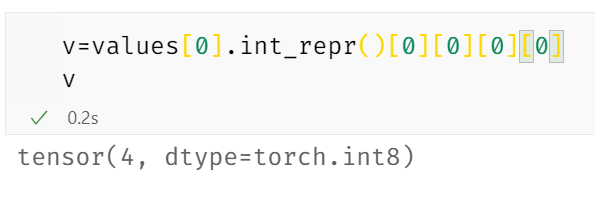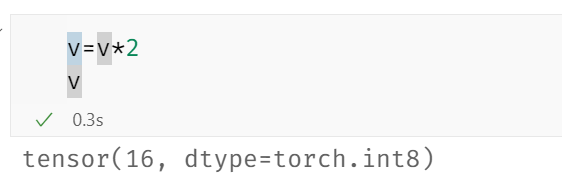# How to change a quant tensor

Hi,
I’m trying to analyze the reliability of a quantized model
But I have a question:
How can I change the respondent value to the model
I change the value directly like what I do in normal models that will not affect the value of the model’s value
like:

``````number.int_repr()=number.int_repr()*2
number.int_repr()
# Don't have any change
number.dequantize()=number.dequantize()*2
number.dequantize()
# Don't change too
``````

Thank you

what kind of changes you want? if you want multiplications by constant then you can just to `quantized_tensor = quantized_tensor * 2` I think. we also have a list of tensor methods defined here: Quantization API Reference — PyTorch master documentation

My fault,
I have read this API Reference, but it can’t help my work
I want to change a number at specified position of matrix
First I get a value from the original matrixThen I change this valueI tried to assign this value to the respondent number
But, it doesn’t affect this matrix at all.Here’s how I get a quant tensor from model
Colab

I see, thanks for the clarification, I think what you need is pytorch/native_functions.yaml at master · pytorch/pytorch · GitHub and pytorch/native_functions.yaml at master · pytorch/pytorch · GitHub which re-assembles quantized Tensor from int_repr, please let me know if it works, thanks

Thank you,
I successfully changed weight in quant models.
But when I use _make_per_tensor_quantized_tensor, it’s changeable.
However, when I use _make_per_channel_quantized_tensor, it’s not changeable

ah, maybe it’s a bug, would you like to file an issue and attach a small repro for it?

Sure, I’m glad to do that.
Here’s issue Quantization: torch._make_per_channel_quantized_tensor doesn’t work well · Issue #68322 · pytorch/pytorch (github.com)
And a few days ago you give me a prototype of FX Graph Mode pytorch/quantized_resnet_test.py at master · pytorch/pytorch (github.com)
But I still don’t know how to inference with CUDA
Does this implementation have some examples

You pretty much can’t do quantized inference with cuda, there are no native quantized cuda kernels atm, our team is working to support lowering to custom backends using Fx to TRT but its not complete yet.

also maybe @jerryzh168 can confirm, but I believe the intended solution was to do:

goal: set int_repr of a quantized tensor x to 3.

``````# x is per channel quantized tensor
x_int = x.int_repr()
x_int=3
x_new = torch._make_per_tensor_quantized_tensor(x_int, x.per_channel_scales(), ... )
``````

not

`x.int_repr()=3`

which i’m fairly sure is not intended to work

2 Likes

here is the example that you can run int8 model in TensorRT: pytorch/quantized_resnet_test.py at master · pytorch/pytorch · GitHub

You are right,
I should change its int_repr() tensor
before _make_per_tensor_quantized_tensor
Thank you